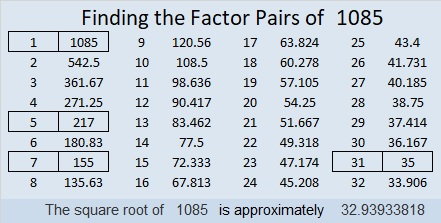# 1085 and Level 5

Here’s another tricky level 5 puzzle for you to solve. Use logic, not guess and check, and you’ll do great!Print the puzzles or type the solution in this excel file: 12 factors 1080-1086

Here are a few facts about the number 1085:

• 1085 is a composite number.
• Prime factorization: 1085 = 5 × 7 × 31
• The exponents in the prime factorization are 1, 1, and 1. Adding one to each and multiplying we get (1 + 1)(1 + 1)(1 + 1) = 2 × 2 × 2 = 8. Therefore 1085 has exactly 8 factors.
• Factors of 1085: 1, 5, 7, 31, 35, 155, 217, 1085
• Factor pairs: 1085 = 1 × 1085, 5 × 217, 7 × 155, or 31 × 35
• 1085 has no square factors that allow its square root to be simplified. √1085 ≈ 32.9393431 × 35 = 1085 means we are  only 4 numbers away from the next perfect square and
33² – 2² = 1085

1085 is the hypotenuse of a Pythagorean triple:
651-868-1085 which is (3-4-5) times 217

1085 looks interesting when it is written using a different base:
It’s 5005 in BASE 6 because 5(6³ + 1) = 1085,
765 in BASE 12 because 7(144) + 6(12) + 5(1) = 1085,
656 in BASE 13 because 6(13²) + 5(13) + 6(1) = 1085
VV in BASE 34 (V is 31 base 10) because 31(34) + 31(1) = 1085, and
it’s V0 in BASE 35 because 31(35) = 1085

This site uses Akismet to reduce spam. Learn how your comment data is processed.